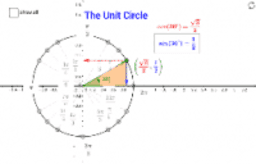Kelly Naylor

•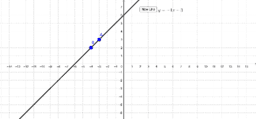Graphing Slope-Intercept Form

Activity

Kelly Naylor

•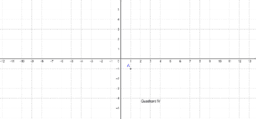GeoGebra Workshop

Book

Kelly Naylor

•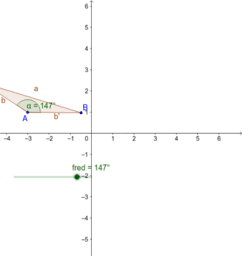Random

Book

Kelly Naylor

•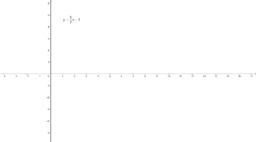fractions

Activity

Kelly Naylor

•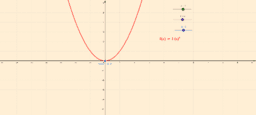Math 2

Book

Kelly Naylor

•Coordinate Plane

Activity

Kelly Naylor

•Math 3

Book

Kelly Naylor

•Mean, Median, Mode

Activity

Kelly Naylor

•Equilateral Triangle Construction

Activity

Kelly Naylor

•Factoring trinomials where a not = 1

Activity

Kelly Naylor

•Factoring trinomials where a = 1

Activity

Kelly Naylor

•Parabolas in Vertex Form

Activity

Kelly Naylor

•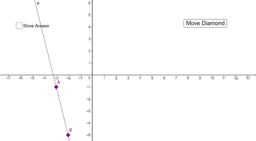Find the Slope-Intercept Equation Given Two Points

Activity

Kelly Naylor

•Max Area of a Triangle

Activity

Kelly Naylor

•